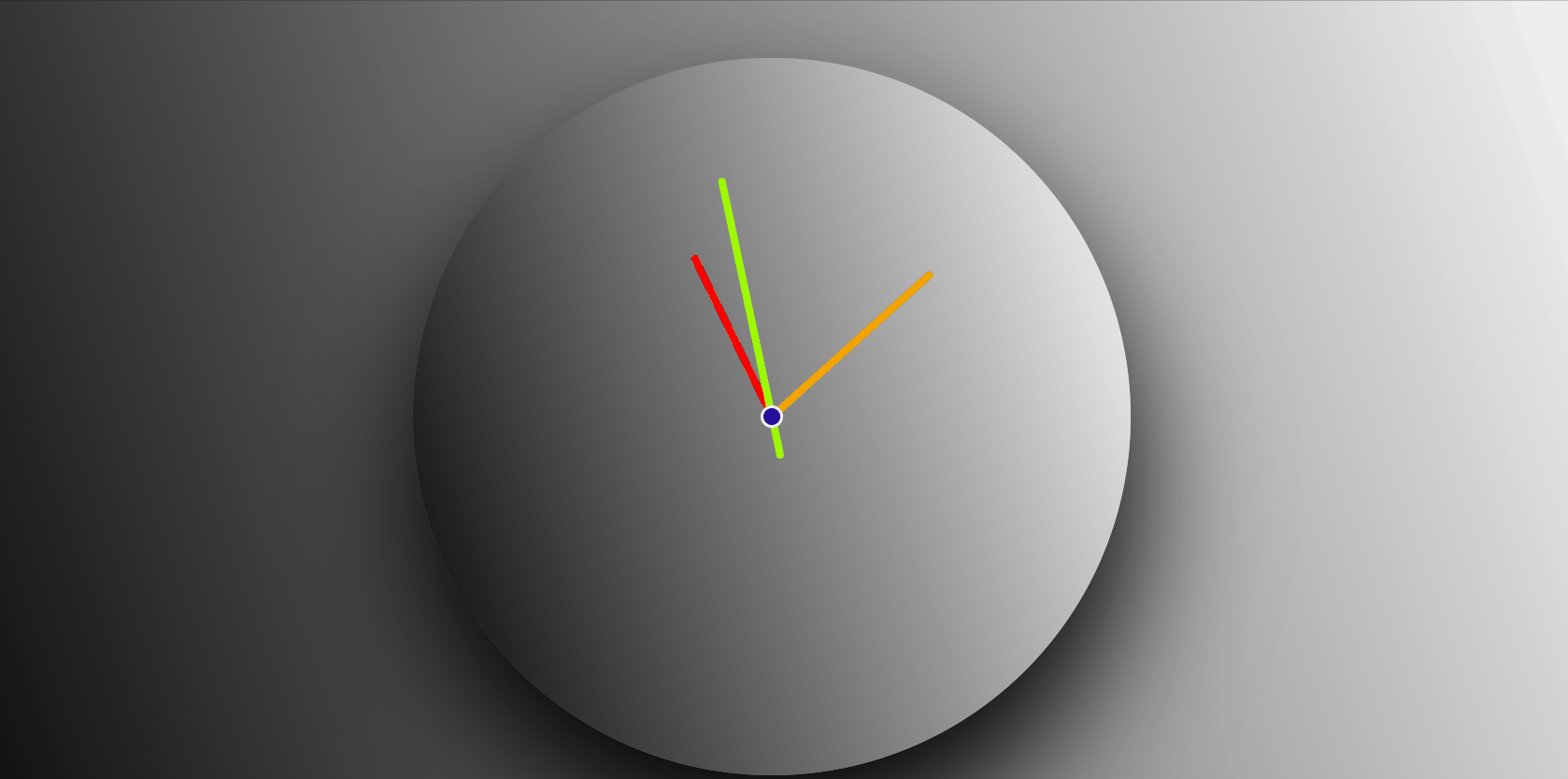GeeksforGeeks App
Open AppBrowser
Continue

# Responsive analog clock using HTML, CSS and Vanilla JavaScript

In this article, we are going to create an Analog Clock. This is mainly based on HTML, CSS & Vanilla JavaScript.

Approach:

1. Create an HTML file in which we are going to add the main div, further on we are adding 4 div tags for an hour, minute, and second hands & for the pin.
2. Create a CSS file for styling our web page and for assigning different lengths to the different hands.
3. Create a JavaScript file for creating a brief logic for the rotation of different clock hands.

The logic for rotation of clock hands:

1. Hour Hand

```For Achieving 12hrs,
hour hand moves 360deg.

i.e.  12hrs   ⇢   360degs

so,    1hr    ⇢   30degs

and, 60mins   ⇢   30degs

so,    1min   ⇢   0.5degs

Total Rotation of hour hand:
(30deg * hrs) + (0.5deg * mins)```

2. Minute Hand

```For Achieving 60mins,
hour hand moves 360deg.

i.e.  60mins   ⇢   360degs

so,    1min    ⇢   6degs

Total Rotation of minute hand:
6deg * mins```

3. Second Hand

```For Achieving 60secs,
hour hand moves 360deg.

i.e.  60secs   ⇢   360degs

so,    1sec    ⇢   6degs

Total Rotation of minute hand:
6deg * secs```

Example:

Code Explanation:

• First, create an HTML file (index.html).
• Now after the creation of our HTML file, we are going to give a title to our webpage using <title> tag. It should be placed inside the <head> section.
• Then we link the CSS file that provides all the styles to our HTML. This is also placed in between the <head> tag.
• Coming to the body section of our HTML code.
• Firstly, create a main div as a clock.
• In that div add 4divs for an hour, minute, and second hands & for the pin.
• At the end of our body add <script> tag which links the JS file with our HTML file.
• The setInterval() function is used for the execution of a function for a specific period of time. For more details click here.
• The Date() function is used for returning today’s date, and current time(hours, minutes, seconds).

## HTML

 ```<``html` `lang``=``"en"``>``   ` `<``head``>``    ``<``title``>Analog Clock``    ``<``link` `rel``=``"stylesheet"` `href``=``"style.css"``>````   ` `<``body``>``    ``<``div` `class``=``"clock"``>``        ``<``div` `class``=``"hr"``>``        ``<``div` `class``=``"min"``>``        ``<``div` `class``=``"sec"``>``        ``<``div` `class``=``"pin"``>``    ```` ` `    ``<``script` `src``=``"index.js"``>````   ` ``

## CSS

 `/* Restoring browser effects */``* {``    ``margin``: ``0``;``    ``padding``: ``0``;``    ``box-sizing: border-box;``    ``;``}`` ` `/* All of the same styling to the body */``body {``    ``height``: ``100``vh;``    ``display``: flex;``    ``justify-``content``: ``center``;``    ``align-items: ``center``;``    ``background-color``: ``#000``;``    ``background-image``: linear-gradient(``            ``70``deg, ``black``, ``white``);``}`` ` `/* Sizing, positioning of main ``    ``dial of the clock */``.clock {``    ``width``: ``40``vw;``    ``height``: ``40``vw;``    ``background-image``: linear-gradient(``                ``70``deg, ``black``, ``white``);``    ``background-``size``: cover;``    ``box-shadow: ``0` `3em` `5.8em``;``    ``border-radius: ``50%``;``    ``position``: ``relative``;``}`` ` `.hr,``.min,``.sec {``    ``width``: ``1%``;``    ``position``: ``absolute``;``    ``top``: ``50%``;``    ``left``: ``50%``;``    ``transform: translate(``-50%``, ``-100%``);``    ``transform-origin: ``bottom``;``    ``z-index``: ``2``;``    ``border-radius: ``2em``;``}`` ` `.pin {``    ``position``: ``absolute``;``    ``top``: ``0``;``    ``left``: ``0``;``    ``right``: ``0``;``    ``bottom``: ``0``;``    ``width``: ``1em``;``    ``height``: ``1em``;``    ``background``: ``rgb``(``38``, ``0``, ``255``);``    ``border``: ``2px` `solid` `#ffffff``;``    ``border-radius: ``10em``;``    ``margin``: ``auto``;``    ``z-index``: ``10``;``}`` ` `/* Different length of different hands of clock */``.hr {``    ``height``: ``25%``;``    ``background-color``: ``#ff0000``;``}`` ` `.min {``    ``height``: ``30%``;``    ``background-color``: ``#ff9900``;``}`` ` `.sec {``    ``height``: ``40%``;``    ``background-color``: ``#99ff00``;``    ``transform-origin: ``50%` `85%``;``}`

## Javascript

 `// Selecting all of the css classes on which``// we want to apply functionalities``const hr = document.querySelector(``'.hr'``)``const min = document.querySelector(``'.min'``)``const sec = document.querySelector(``'.sec'``)`` ` `// Setting up the period of working``setInterval(() => {`` ` `    ``// Extracting the current time ``    ``// from DATE() function``    ``let day = ``new` `Date()``    ``let hour = day.getHours()``    ``let minutes = day.getMinutes()``    ``let seconds = day.getSeconds()`` ` `    ``// Formula that is explained above for ``    ``// the rotation of different hands``    ``let hrrotation = (30 * hour) + (0.5 * minutes);``    ``let minrotation = 6 * minutes;``    ``let secrotation = 6 * seconds;`` ` `    ``hr.style.transform =``        ```translate(-50%,-100%) rotate(\${hrrotation}deg)```    ``min.style.transform =``        ```translate(-50%,-100%) rotate(\${minrotation}deg)```    ``sec.style.transform =``        ```translate(-50%,-85%) rotate(\${secrotation}deg)```});`Responsive analog clock using HTML, CSS and Vanilla JavaScript

My Personal Notes arrow_drop_up
Related Tutorials